Publication date: 08/13/2020

## Parameterizations for Distributions

This section gives the density functions f for the distributions used in the Process Capability platform. It also gives expected values and variances for all but the Johnson and SHASH distributions.

#### Normal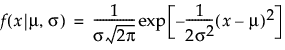,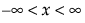,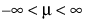,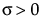E(X) = μ

Var(X) = σ2

#### Beta,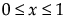,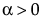,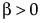E(X) =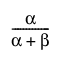Var(X) =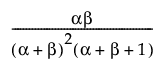where B(·) is the Beta function.

#### Exponential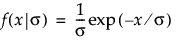,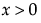,E(X) = σ

Var(X) = σ2

#### Gamma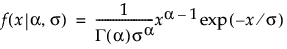,,,E(X) = ασ

Var(X) = ασ2

where Γ(·) is the gamma function.

#### Johnson

Johnson Su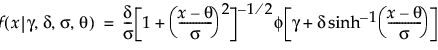,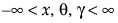,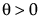,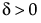Johnson Sb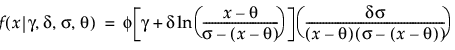,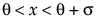,Johnson Sl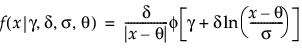,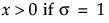,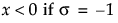where φ(·) is the standard normal probability density function.

#### Lognormal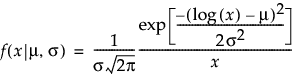,,,E(X) =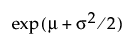Var(X) =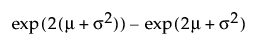#### Mixture of Normals

The Mixture of 2 Normals and Mixture of 3 Normals options for Distribution share the following parameterization: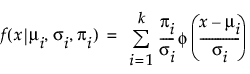E(X) =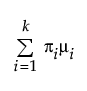Var(X) =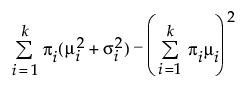where μi, σi, and πi are the respective mean, standard deviation, and proportion for the ith group, and φ(·) is the standard normal probability density function. For the Mixture of 2 Normals, k is equal to 2. For the Mixture of 3 Normals distribution, k is equal to 3. A separate mean, standard deviation, and proportion of the whole is estimated for each group in the mixture.

#### SHASH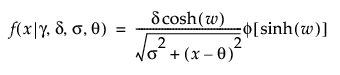,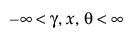, 0 < δ, 0 < σ

where

φ(·) is the standard normal pdf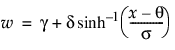Note: When γ = 0 and δ = 1, the SHASH distribution is equivalent to the normal distribution with location θ and scale σ.

#### Weibull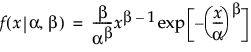,,E(X) =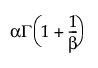Var(X) =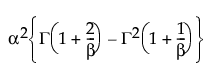where Γ(·) is the gamma function.